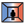##MRT Calculator - [source code]Use this component calculate Mean Radiant Temperature (MRT) given a set of temperatures and corresponding view factors. This component will check to be sure view factors add to 1 and will use the following formula: MRT = (V1T1^4 + V2T2^4 + ...) ^ (1/4) Where V corresponds to a view factor and T corresponds to a temperature. -

#### Inputs

• ##### temperatures [Required]
A list of radiant temperatures in Celcius that correspond to view factors below.
• ##### viewFactors [Required]
A list of viewFactors that correspond to the temperatures above. These should sum to 1.

#### Outputs

• ##### MRT
The Mean Radiant Temperature that results from the input temperatures and view factors.

Check Hydra Example Files for MRT Calculator